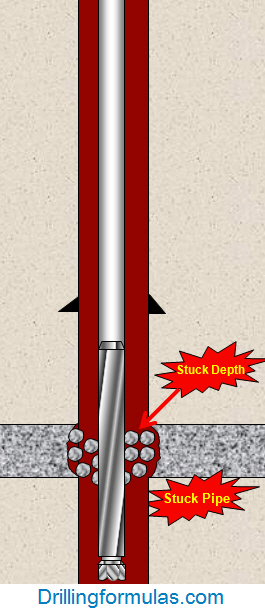# Determine how many feet of free pipe without free pipe constant table

You may sometimes find the table for Free Point Constant for your stuck pipe calculation so you use the formula from the previous post to determine Free Point Constant and apply it into free pipe formula to determine where the depth of stuck pipe is.Stuck Pipe Calculation – Stuck Depth

The steps of calculation are as follows:

1 ) Determine the free point constant (FPC) by this following formula:

FPC = As x 2500

where: As = pipe wall cross sectional area, sq in.

As = (OD2-ID2) x 0.7854

OD and ID are inch unit

2) Determine depth of stuck pipe by this following formula:

Depth of stuck pipe = (Pipe stretch in inch x free point constant (FPC)) ÷ Pull force in thousands of pounds

Please see the example below to demonstrate you how to apply those 2 formulas above into real drilling operation.

Example#2: Determine the free point constant (FPC) and the depth the pipe is stuck using the following information:

3-1/2” tubing # 9.5 lb/ft

3-1/2” tubing ID = 2.992 inch

20 inch of stretch with 25 Klb of pulling force

a) Determine free point constant (FPC):

FPC = (3.52 – 2.9922) x 0.7854 x 2500

FPC = 6475.5

b) Determine the depth of stuck pipe:

Feet of free pipe (ft) = (20 inch x 6475.5) ÷ 25

** 25 is the kilo-force to pull to 20 inch **

Feet of free pipe (ft) = 5,180 ft

Please find the Excel sheet used for calculating depth of stuck pipe.

Share the joyWorking in the oil field and loving to share knowledge.

### 4 Responses to Determine how many feet of free pipe without free pipe constant table

1.Chris Cabral says:

In the calculations above in “Determine how many feet of free pipe without free pipe constant table” b) determine length of free pipe….pipe stretch(20 inches) x free pipe constant(6475.5) divided by 25 feet..where does the 25feet come from?

•DrillingFormulas.Com says:

In my blog, it may lead confusion to you because I put feet after 25. It means that after all calculation is done, the unit that you will get is feet. 25 is Kilo lb force pull in order to get 20 inch stress.

2.Ricardo says:

How do I make free point calculations for horizontal wells

•DrillingFormulas.Com says:

For the horizontal wells, the free point calculation may not be really accurate because it has a lot of drag effect because the string lays on the low side of the well.

Regards,
Shyne.

This site uses Akismet to reduce spam. Learn how your comment data is processed.